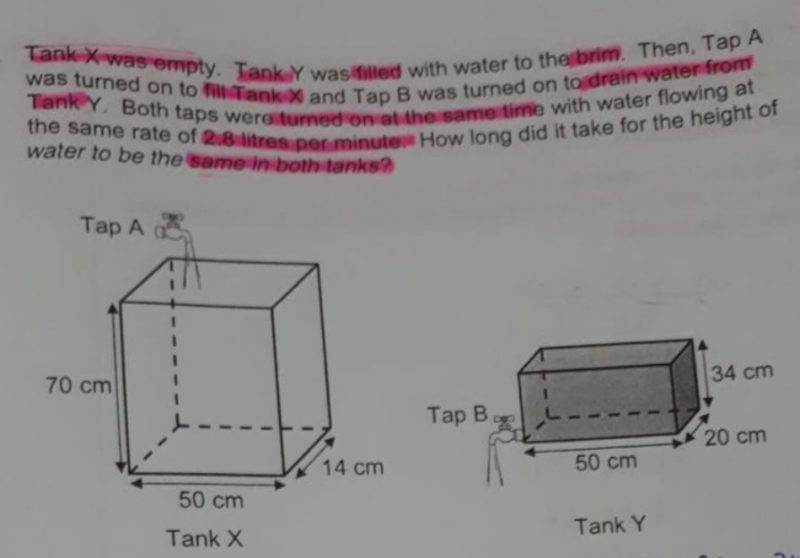# QuestionHi, we need help with this qn. Tq!

Base area for X = 50 X 14 = 700 cm2
Base area for Y = 50 X 20 = 1000 cm2

Rise in cm rate for X = 2800/700 = 4cm/min
Drop in cm rate for Y = 2800/1000 = 2.8 cm/min

Time taken when height is equal
4t = 34 – 2.8t
6.8t = 34
t = 5 min ###

Thank u! Can u explain how did u get 4t and 2.8t?

I have calculated that the rise in water for Tank X is 4 cm/min.  And drop in height for  Tank Y to be 2.8 cm/min.

It is flow rate divide by the base area,

• Rise in cm rate for X = 2800/700 = 4cm/min
• Drop in cm rate for Y = 2800/1000 = 2.8 cm/min

“t” is the time in minutes for the height of both tanks to be equal . The height is expressed as 4t for tank X and 34 -2.8t for tank Y.

4t = 34 – 2.8t

6.8t = 34

t = 5 mins##

0 Replies 1 Like

2.8 litres = 2800 cubic cm
In 1 min, the height of Tank:
X increases by : 2800/(50 x 14) = 4
Y decreases by : 2800/(50 x 20) = 2.8

34/(4 + 2.8) = 5
or
(4 x time) = 34 – (2.8 x time)
time = 5

Ans : 5 min.

0 Replies 0 Likes